Courses

# Test: Equilibrium 3 - From Past 28 Years Questions

## 30 Questions MCQ Test Chemistry for JEE | Test: Equilibrium 3 - From Past 28 Years Questions

Description
This mock test of Test: Equilibrium 3 - From Past 28 Years Questions for NEET helps you for every NEET entrance exam. This contains 30 Multiple Choice Questions for NEET Test: Equilibrium 3 - From Past 28 Years Questions (mcq) to study with solutions a complete question bank. The solved questions answers in this Test: Equilibrium 3 - From Past 28 Years Questions quiz give you a good mix of easy questions and tough questions. NEET students definitely take this Test: Equilibrium 3 - From Past 28 Years Questions exercise for a better result in the exam. You can find other Test: Equilibrium 3 - From Past 28 Years Questions extra questions, long questions & short questions for NEET on EduRev as well by searching above.
QUESTION: 1

### The compound whose aqueous solution has the highest pH is 

Solution:

NaCl is a salt of strong acid and strong base hence its aqueous solution will be neutral ie pH = 7. NaHCO3 is an acidic salt hence pH < 7 . Na2CO3 is a salt of weak acid and strong base. Hence its aqueous solution will be strongly basic ie. pH > 7.
NH4Cl is salt of weak base and strong acid, hence its aqueous solution will be strongly acidic i.e. pH < 7.

QUESTION: 2

### K1 and K2 are equilibrium constant for reactions (1) and (2)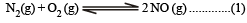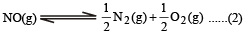Solution:

For reation (1)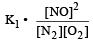and for reaction (2)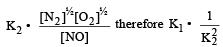QUESTION: 3

### Aqueous solution of acetic acid contains 

Solution:

An aqueo us solution of acetic acid dissociates as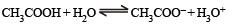QUESTION: 4

0.1 M solution of which one of these substances will be basic ? 

Solution:

Sodium borate is a salt of strong base (NaOH) and weak acid (H3BO3). Hence its aqueous solution will be basic

QUESTION: 5

Which one of the following information can be obtained on the basis of Le Chatelier principle?

Solution:

According to Le-ch atelier's principle" whenever a constraint is applied to a system in equilibrium, the system tends to readjust so as to nullify the effect of the constraint.

QUESTION: 6

In which of the following solvents, AgBr will have the highest solubility ? 

Solution:

AgBr has the highest solubility in 10-3 M NH4OH . All other solven ts will dissolve AgBr poorly. Moreover bromides of Ag+, Hg22+ and Cu22+ are water insoluble

QUESTION: 7

According to Le-chatelier ’s principle, adding heat to a solid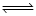liquid equilibrium will cause the

Solution: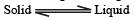It is an endothermic process. So when temperature is raised, more liquid is formed.
Hence adding heat will shift the equilbrium in the forward direction.

QUESTION: 8

In which of the following the solubility of AgCl will be minimum ? 

Solution:

In presence of the given salts, the solubility of AgCl decreases due to common ion effect.

QUESTION: 9

Which of the following is most soluble ? 

Solution:

For Bi2S3 .   Ksp– = (2s)2.(3s)3
= 4s2. 27s3 = 108s5

or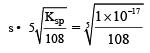For MnS. Ksp = s2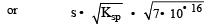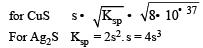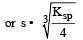thus MnS has maximum solubility.

QUESTION: 10

The pH value of a 10 M solution of HCl is 

Solution:

Molarity (M) = 10M. HCl is a str ong acid and it is completely dissociated in aqueous solutions as :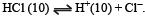So, for every moles of HCl, there is one H+.
Therefore [H+] = [HCl] or [H+] = 10. pH = – log[H+] = – log  = – 1.

QUESTION: 11

The rate constant for forward and backward reaction of hydrolysis of ester are 1.1x10-2 and 1.5x10-3 per minute respectively. Equilibrium constant for the reaction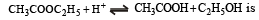Solution:

Rate constant of forward reaction (Kf) = 1.1 × 10–2 and rate constant of backward reaction (Kb) = 1.5 × 10–3 per minute. Equilibrium constant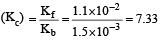QUESTION: 12

Th e pH value of blood does not appreciably change by a small addition of an acid or a base, because the blood 

Solution:

The buffer system present in serum is H2CO3 + NaHCO3 and as we known that a buffer solution resist the change in pH therefore pH value of blood does not change by a small addition of an acid or a base.

QUESTION: 13

If α is the fraction of HI dissociated at equilibrium in the reaction,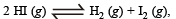starting with 2 moles of HI, the total number of moles of reactants and products at equilibrium are

Solution:

According to equation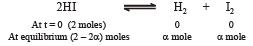Total moles at equilibrium = 2 – 2α +α+α = 2 mole

QUESTION: 14

The equilibrium con stant for the reaction A2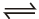2A at 500 K and 700 K are 1 × 10–10 and 1× 10–5 respectively. The given reaction is

Solution: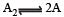Equilibrium constant is given by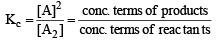Since the value given is very small, hence conc. of products is less. It means the reaction is slow.

QUESTION: 15

The solubility products of CuS, Ag2S and HgS are 10–31, 10–44, 10–54 respectively. The solubilities of these sulphides are in the order

Solution: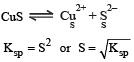For Binary salts like CuS & HgS, solubilty,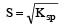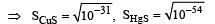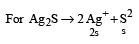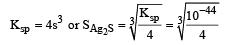∴ The order is CuS > Ag2S > HgS

QUESTION: 16

A physician wishes to prepare a buffer solution of pH = 3.58 that efficiently resists a change in pH yet contains only small concentrations of the buffering agents. Which one of the following weak acids together with its sodium salt would be the best to use ? 

Solution: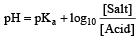For small concentration of buffering agent and for maximum buffer capacity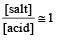∴ pH = pKa

QUESTION: 17

In a two-step exothermic reaction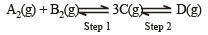Steps 1 and 2 are favoured respectively by 

Solution: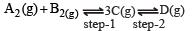since the steps 1 and 2 are exothermic hence low temprature will favour both the reactions.
In step - 1 moles are increasing hence low pressure will favour it. In step 2 moles are decreasing, hence high pressure will favour it.

QUESTION: 18

If K1 and K2 are the respective equilibrium constants for the two reactions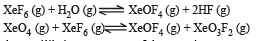the equilibrium constant of the reaction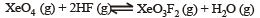will be

Solution:

For the reaction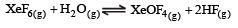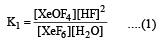and for the reaction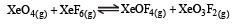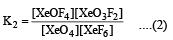For reaction :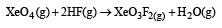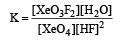∴   From eq. no. (1) and (2)
K = K2/ K1

QUESTION: 19

Among boron trifluoride, stannic chloride and stannous chloride, Lewis acid is represented by

Solution:

Lewis acid is that compound which have electron deficiency. eg. BF3, SnCl2.

QUESTION: 20

What is the H+ ion concentration of a solution prepared by dissolving 4 g of NaOH (Atomic weight of Na = 23 amu) in 1000 ml? 

Solution:

No. of moles of NaOH =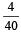= 0.1

[Molecular weight of NaOH = 40]

No. of moles of OH = 0.

Concentration of OH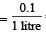= 0.1Mole /L

As we know that, [H+] [OH ] = 1014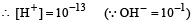QUESTION: 21

The solubility product of a sparingly soluble salt BA2 is 4 × 10–12. The solubility of BA2 is

Solution: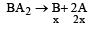Solubility product = [x]  [2x]2 = 4x3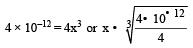∴   x = 10–4

QUESTION: 22

A base when dissolved in water yields a solution with a hydroxyl ion concentration of 0.05 mol litre–1.The solution is 

Solution:

Given : Hydroxyl ion concentration [OH] = 0.05 mol L–1. We know that the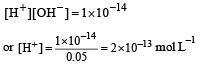We also know that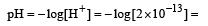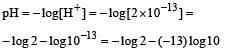Since the value of pH > 7, therefore the solution is basic.

QUESTION: 23

Value of KP in the reaction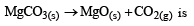Solution:

MgCO3(s) → MgO(s) + CO2(g) MgO & MgCO3 are solid and they donot exert any pressure and hence only pressure exerted is by CO2. Therefore KP = PCO2

QUESTION: 24

Conjugate acid of NH-2 is : 

Solution:

Because NH3 after losing a proton (H+) gives NH2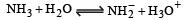(Conjugate acid-base pair differ only by a proton)

QUESTION: 25

For dibasic acid correct order is 

Solution:

In polyprotic acids the loss of second proton occurs much less readily than the first.
Usually the Ka values for successive loss of protons from these acids differ by at least a factor of 10–3 i.e.,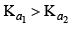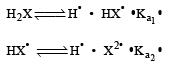QUESTION: 26

Which of the following statements about pH and H+ ion concentration is incorrect? 

Solution:

CH3COOH is weak acid while NaOH is strong base, so one equivalent of NaOH can not be neutralized with one equivalent of CH3COOH.
Hence the solution of one equivalent of each does not have pH value as 7. Its pH will be towards basic side as NaOH is a strong base hence conc. of OH will be more than the conc. of H+.

QUESTION: 27

Solubility of a M2S salt is 3.5 × 10–6, then its solubility product will be 

Solution: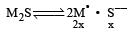Solubility product = (2x)2 (x) = 4x3 = 4 (3.5 × 10–6)3  = 1.7 × 10–16

QUESTION: 28

Ionisation constant of CH3COOH is 1.7 × 10–5 if concentration of H+ ions is 3.4 × 10–4M, then find out initial concentration of CH3COOH molecules 

Solution: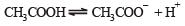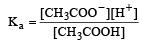Given that,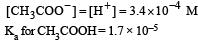CH3COOH is weak acid, so in it [CH3COOH] is equal to initial concentration. Hence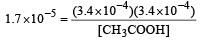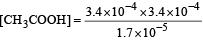= 6.8 × 10–3M

QUESTION: 29

In HS, I, RNH2 and NH3, order of proton accepting tendency will be 

Solution:

Strong base has higher tendency to accept the proton. Increasing order of base and hence the order of accepting tendency of proton is I- < HS- < NH3 < RNH2

QUESTION: 30

For the reaction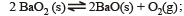ΔH = +ve. In equilibrium condition, pressure of O2 is dependent on 

Solution:

For the reaction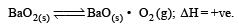At equilibrium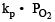[For solid and liquids concentration term is taken as unity]
Hence, the value of equilibrium constant depends only upon partial pressure of O2.
Further on increasing temperature formation of O2 increases as this is an endothermic reaction.